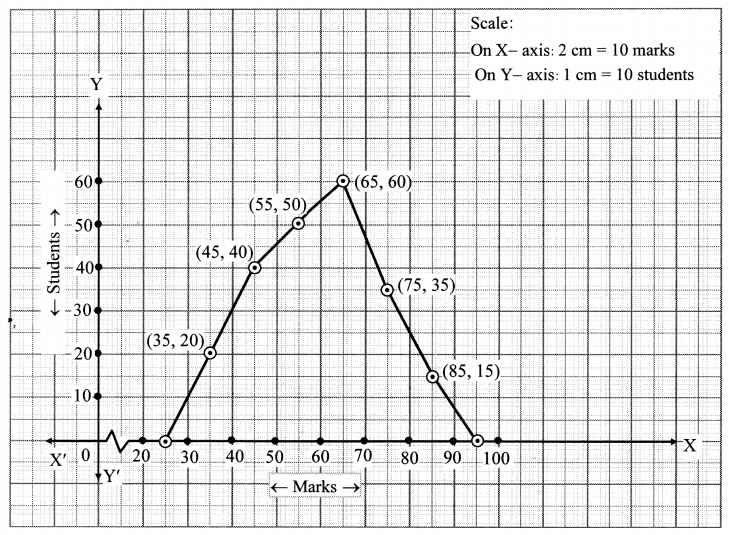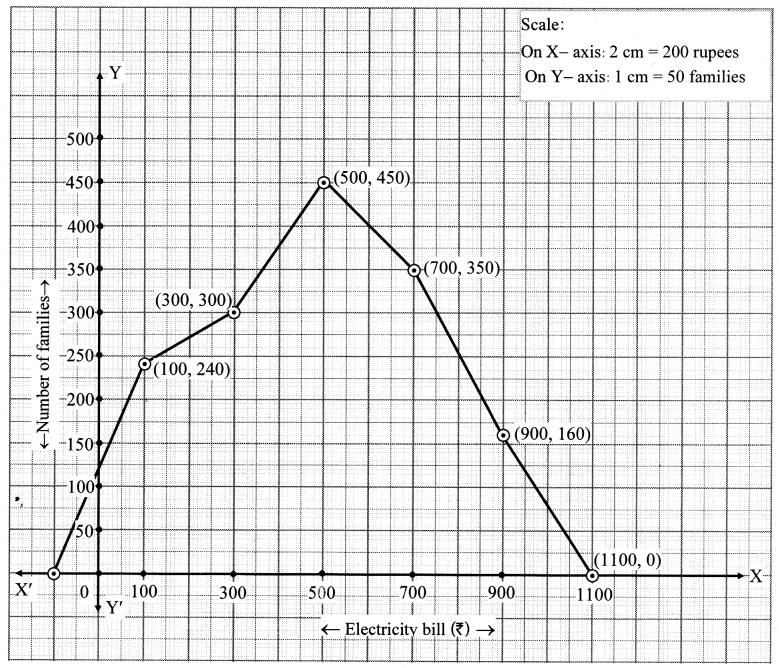# Maharashtra Board 10th Class Maths Part 1 Practice Set 6.5 Solutions Chapter 6 Statistics

Balbharti Maharashtra State Board Class 10 Maths Solutions covers the Practice Set 6.5 Algebra 10th Class Maths Part 1 Answers Solutions Chapter 6 Statistics.

## Practice Set 6.5 Algebra 10th Std Maths Part 1 Answers Chapter 6 Statistics

Question 1.
Observe the following frequency polygon and write the answers of the questions below it.
i. Which class has the maximum number of students?
ii. Write the classes having zero frequency.
iii. What is the class mark of the class, having frequency of 50 students?
iv. Write the lower and upper class limits of the class whose class mark is 85.
v. How many students are in the class 80 – 90?Solution:
i. The class 60 – 70 has the maximum number of students.
ii. The classes 20 – 30 and 90 – 100 have frequency zero.
iii. The class mark of the class having 50 students is 55.
iv. The lower and upper class limits of the class having class mark 85 are 80 and 90 respectively.
v. There are 15 students in the class 80 – 90.

Question 2.
Show the following data by a frequency polygon.Solution:Question 3.
The following table shows the classification of percentages of marks of students and the number of students. Draw a frequency polygon from the table.Solution: